## Example Questions

### Example Question #1 : How To Find The Volume Of A Sphere

How much does the volume of a sphere increase if its radius is increased by 50%?

Possible Answers:

237.5%

50%

0.3375%

150%

337.5%

Correct answer:

237.5%

Explanation:

Recall the equation for the volume of a sphere:

V = (4/3)πr3

If we increase the radius by 50%, we can represent the new radius as being equal to r + 0.5r = 1.5r.

Replace this into the equation for the volume and simplify:

V2 = (4/3)π(1.5r)3 = (4/3)π(3.375r3)

Rewrite this so that you can compare the two volumes:

V= 3.375 * (4/3)πr3 = 3.375 * [(4/3)πr3]

This is the same as:

V2 = 3.375 * V

This means that the new volume is 337.5% of the original.  However, note that the question asked for the increase, which would be an increase by 237.5%.

### Example Question #2 : How To Find The Volume Of A Sphere

A cube weighs 216 grams. If you carve a sphere out of the cube such that the diameter of the sphere is equal to one of the sides of the square, how many grams is the weight of the resulting sphere?

Possible Answers:

144π

36π

216π

288π

Correct answer:

36π

Explanation:

Remember that the weight of an object is analogous to the volume. Since the weight of the sphere is 216, the volume of the sphere is proportional to 216. Remember the equation for volume of a sphere:

V = a * a * a = 216

Take the cube root of 216 to find that the length of one of the cubes is proportional to 6. According to the question, one of the sides of the cube is equivalent to the diameter of the sphere.

Thus d = 6 and r = d/2 = 3 for the sphere.

Remember the volume equation for a sphere:

V = 4/3 * π * r3

Plug in r = 3 to find V = 36π

### Example Question #3 : How To Find The Volume Of A Sphere

What is the volume of a sphere with a radius of 3?

Possible Answers:

36π

24π

48π

112π

96π

Correct answer:

36π

Explanation:

Volume of a sphere = 4/3 * πr3 = 4/3 * π * 3= 36π

### Example Question #1 : How To Find The Volume Of A Sphere

How many times greater is the volume of a sphere with radius of 3 than the volume of a sphere with radius of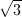?

Possible Answers: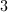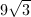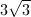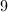Correct answer:Explanation:

The formula for the volume of a sphere is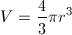.

Use this to find the volume of each sphere, then take the quotient of the two volumes to determine the relationship between the two.

For the first sphere,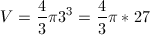.

For the second sphere,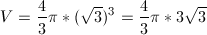.

Since both volumes have factors of 4/3 and pi, we'll ignore those and divide by the remaining factors. Keep in mind that the volume of the first sphere must be in the numerator due to the wording of the question.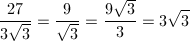### All GRE Math Resources